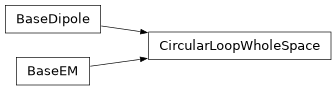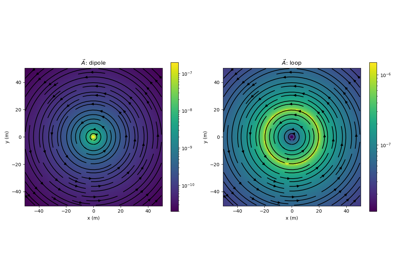# geoana.em.static.CircularLoopWholeSpace#class geoana.em.static.CircularLoopWholeSpace(radius=0.5641895835477563, current=1.0, **kwargs)#

Class for a circular loop of static current in a wholespace.

The CircularLoopWholeSpace class is used to analytically compute the fields and potentials within a wholespace due to a circular loop carrying static current.

Parameters
currentfloat

Electrical current in the loop (A). Default is 1.

Radius of the loop (m). Default is $$\pi^{-1/2}$$ so that the loop has a default dipole moment of 1 $$A/m^2$$.

Attributes

 current Current in the loop in Amps epsilon Dielectric permittivity in F/m location Location of the dipole mu Magnetic permeability in H/m orientation Orientation of the dipole as a normalized vector radius Radius of the loop in meters sigma Electrical conductivity in S/m

Methods

 Cross products between a gridded set of vectors and the orientation of the source. distance(xyz) Scalar distance from dipole to a set of gridded xyz locations Dot product between the orientation of the source and a gridded set of vectors. magnetic_field(xyz[, coordinates]) Compute the magnetic field for the current loop in a wholespace. magnetic_flux_density(xyz[, coordinates]) Compute the magnetic flux density for the current loop in a wholespace. Vector distance to a set of gridded xyz locations. vector_potential(xyz[, coordinates]) Compute the vector potential for the static loop in a wholespace.

## Galleries and Tutorials using geoana.em.static.CircularLoopWholeSpace#Magnetostatic Vector Potentials: Dipole and Loop Sources

Magnetostatic Vector Potentials: Dipole and Loop Sources